# Binormal

(diff) ← Older revision | Latest revision (diff) | Newer revision → (diff)

The straight line passing through a pointof a curve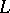perpendicular to the osculating plane toat. If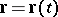is a parametrization of, then the vector equation of the binormal atcorresponding to the valueof the parameterhas the form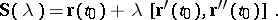This definition holds for space curves for whichdoes not depend linearly on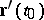, i.e. the curvature should not vanish.
For curves in a higher-dimensional Euclidean space, the binormal is generated by the second normal vector in the Frénet frame (cf. Frénet trihedron), which is perpendicular to the plane spanned byand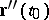and depends linearly on(cf. [a1]).